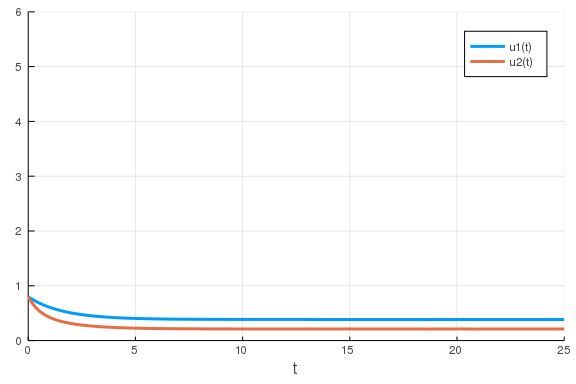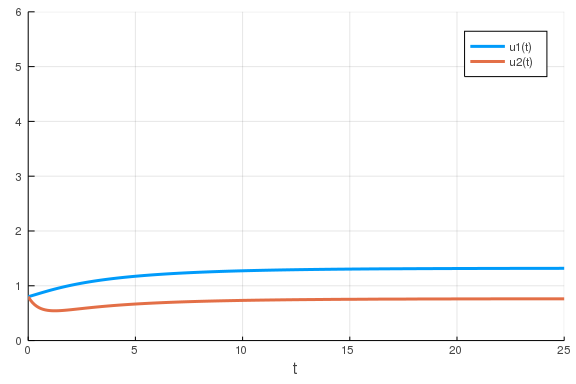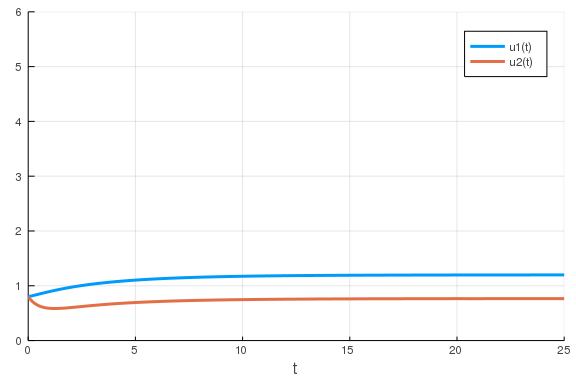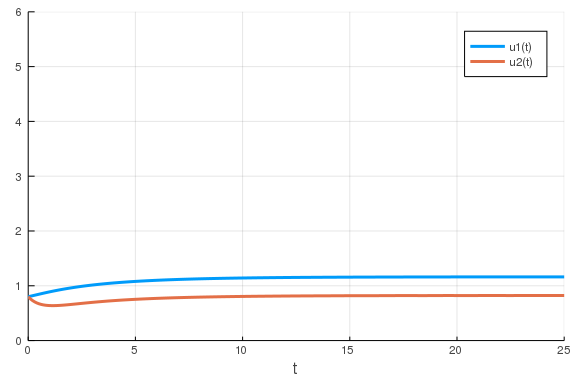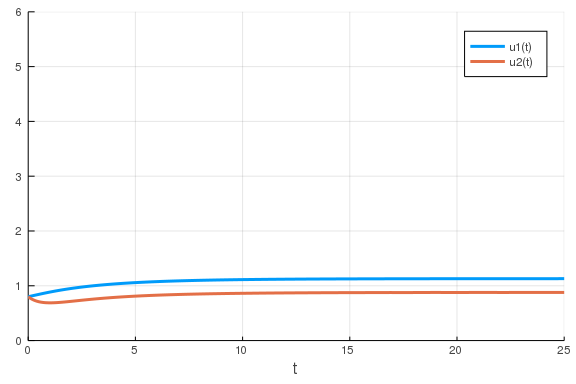# Mixing Differential Equations and Machine Learning

## Neural and Universal Ordinary Differential Equations

The starting point for our connection between neural networks and differential equations is the neural differential equation. If we look at a recurrent neural network:

$x_{n+1} = x_n + NN(x_n)$

in its most general form, then we can think of pulling out a multiplication factor $h$ out of the neural network, where $t_{n+1} = t_n + h$, and see

$x_{n+1} = x_n + \Delta t NN(x_n)$

$\frac{x_{n+1} - x_n}{h} = NN(x_n)$

and if we send $h \rightarrow 0$ then we get:

$x' = NN(x)$

which is an ordinary differential equation. Discretizations of ordinary differential equations defined by neural networks are recurrent neural networks!

### Training Ordinary Differential Equations

For the full overview on training neural ordinary differential equations, consult the 18.337 notes on the adjoint of an ordinary differential equation for how to define the gradient of a differential equation w.r.t to its solution. These details we will dig into later in order to better control the training process, but for now we will simply use the default gradient calculation provided by DiffEqFlux.jl in order to train systems.

As a starting point, we will begin by "training" the parameters of an ordinary differential equation to match a cost function. Recall that this is what we did in the last lecture, but in the context of scientific computing and with standard optimization libraries (Optim.jl). Now let's rephrase the same process in terms of the Flux.jl neural network library and "train" the parameters.

First, let's define our example. We will once again use the Lotka-Volterra system:

using OrdinaryDiffEq
function lotka_volterra(du,u,p,t)
x, y = u
α, β, δ, γ = p
du = dx = α*x - β*x*y
du = dy = -δ*y + γ*x*y
end
u0 = [1.0,1.0]
tspan = (0.0,10.0)
p = [1.5,1.0,3.0,1.0]
prob = ODEProblem(lotka_volterra,u0,tspan,p)
sol = solve(prob,Tsit5())
test_data = Array(solve(prob,Tsit5(),saveat=0.1))
using Plots
plot(sol)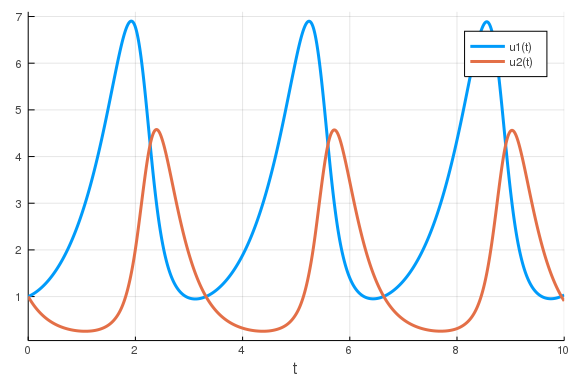Next we define a "single layer neural network" that uses the concrete_solve function that takes the parameters and returns the solution of the x(t) variable. concrete_solve is a function over the DifferentialEquations solve that is used to signify which backpropogation algorithm to use to calculate the gradient. It is a function of the parameters (and optionally one can pass an initial condition). We use it as follows:

using Flux, DiffEqFlux
p = [2.2, 1.0, 2.0, 0.4] # Initial Parameter Vector

function predict_adjoint() # Our 1-layer neural network
Array(concrete_solve(prob,Tsit5(),u0,p,saveat=0.1,abstol=1e-6,reltol=1e-5))
end

predict_adjoint (generic function with 1 method)


Next we choose a loss function. Our goal will be to find parameter that make the Lotka-Volterra solution constant x(t)=1, so we defined our loss as the squared distance from 1:

loss_adjoint() = sum(abs2,predict_adjoint() - test_data)

loss_adjoint (generic function with 1 method)

iter = 0
cb = function () #callback function to observe training
global iter += 1
if iter % 50 == 0
# using remake to re-create our prob with current parameters p
pl = plot(solve(remake(prob,p=p),Tsit5(),saveat=0.0:0.1:10.0),lw=5,ylim=(0,8))
display(scatter!(pl,0.0:0.1:10.0,test_data',markersize=2))
end
end

# Display the ODE with the initial parameter values.
cb()

p = [2.2, 1.0, 2.0, 0.4]

data = Iterators.repeated((), 300)
Flux.train!(loss_adjoint, Flux.params(p), data, opt, cb = cb)

32.33895743168287
11.626035761790217
3.8279028122170042
1.0186999772431977
0.23052507651141854
0.04612786274097307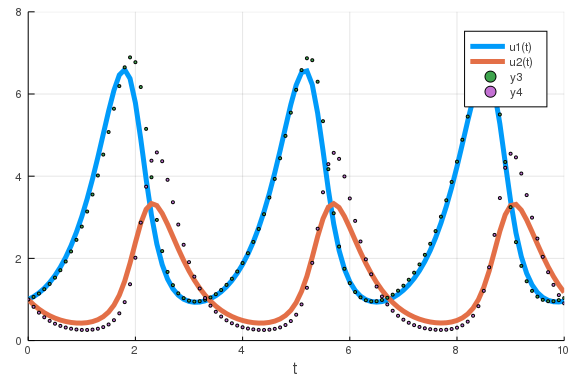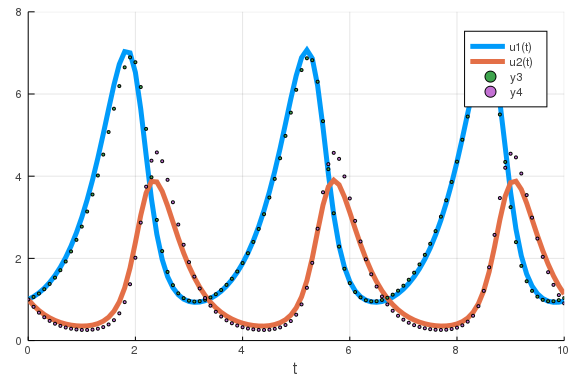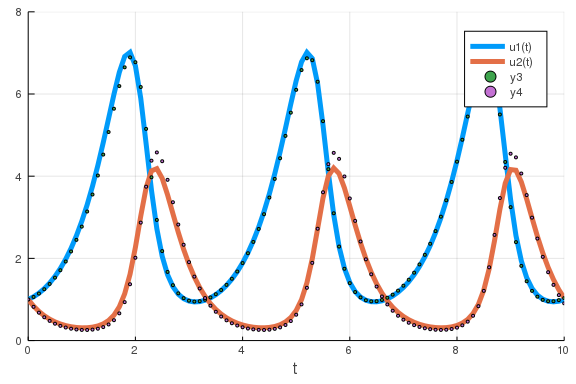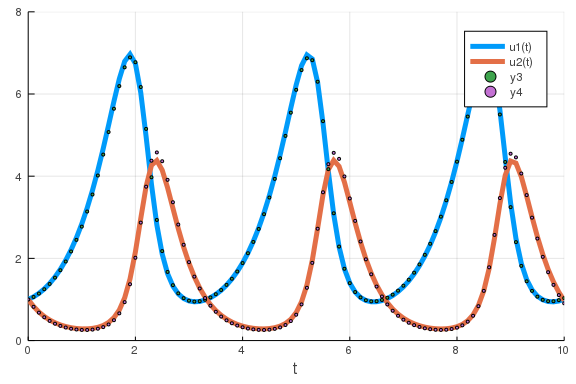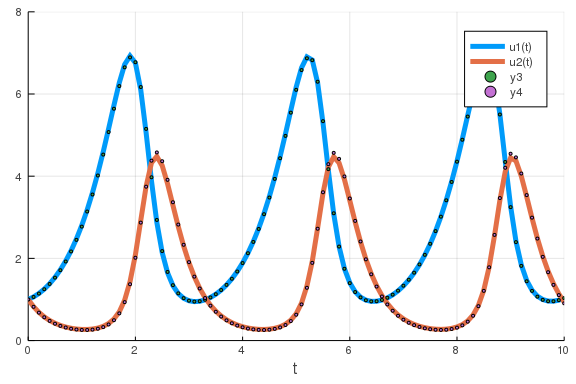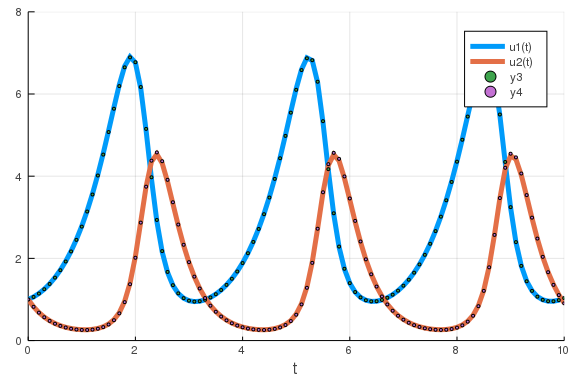and then use gradient descent to force monotone convergence:

data = Iterators.repeated((), 300)
opt = Descent(0.00001)
Flux.train!(loss_adjoint, Flux.params(p), data, opt, cb = cb)

0.042492548405884414
0.04070653643244374
0.03900607872060557
0.03738347043648228
0.03583475604907156
0.034356433857518395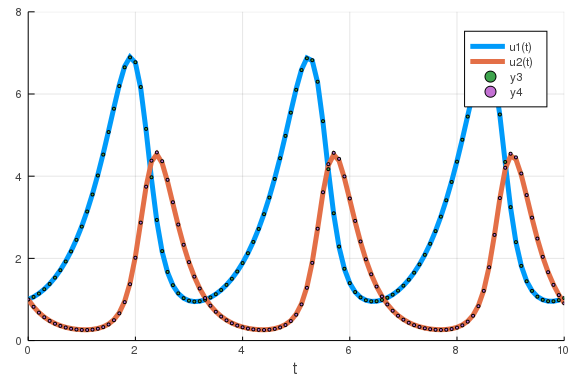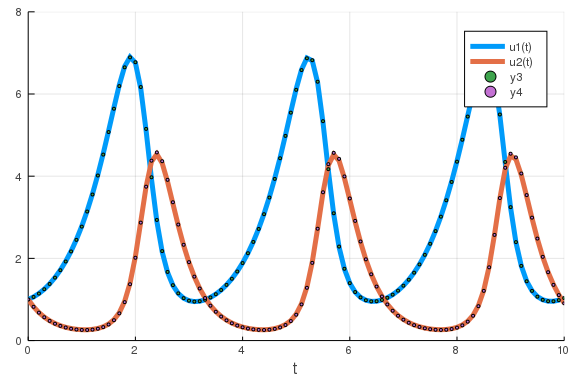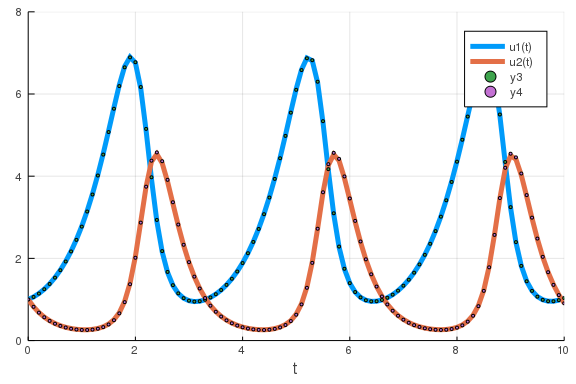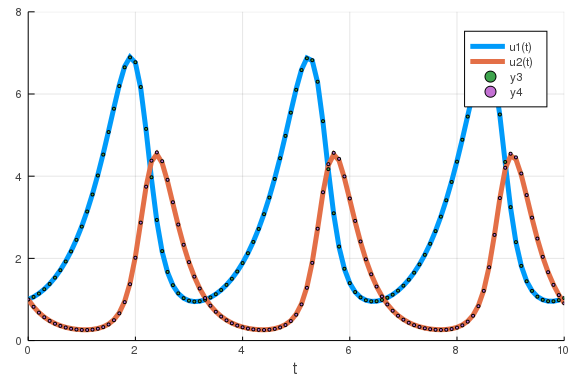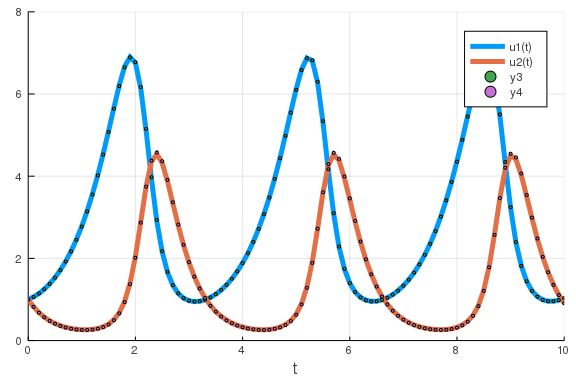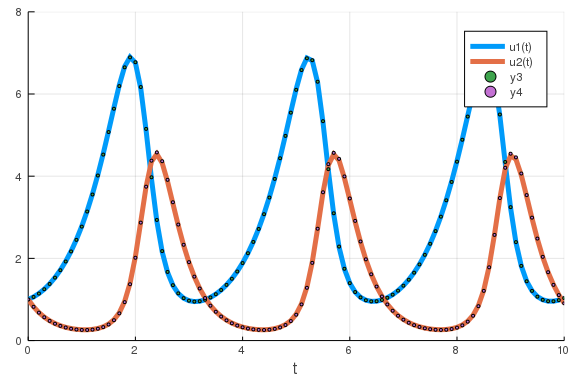### Defining and Training Neural Ordinary Differential Equations

Defining a neural ODE is the same as defining a parameterized differential equation, except here the parameterized ODE is simply a neural network.

Let's try to match the following data:

u0 = Float32[2.; 0.]
datasize = 30
tspan = (0.0f0,1.5f0)

function trueODEfunc(du,u,p,t)
true_A = [-0.1 2.0; -2.0 -0.1]
du .= ((u.^3)'true_A)'
end
t = range(tspan,tspan,length=datasize)
prob = ODEProblem(trueODEfunc,u0,tspan)
ode_data = Array(solve(prob,Tsit5(),saveat=t))

2×30 Array{Float32,2}:
2.0  1.9465    1.74178  1.23837  0.577126  …  1.40688   1.37022   1.29214
0.0  0.798831  1.46473  1.80877  1.86465      0.451381  0.728698  0.972098


and do so with a "knowledge-infused approach". To do so, assume that we knew that the defining ODE had some cubic behavior. We can define the following neural network which encodes that physical information:

dudt = Chain(x -> x.^3,
Dense(2,50,tanh),
Dense(50,2))

Chain(#3, Dense(2, 50, tanh), Dense(50, 2))


Now we want to define and train the ODE described by that neural network. To do so, we will make use of the helper functions destructure and restructure which allow us to take the parameters out of a neural network into a vector and rebuild a neural network from a parameter vector. Using these functions, we would define the following ODE:

p,re = Flux.destructure(dudt)
dudt2_(u,p,t) = re(p)(u)
prob = ODEProblem(dudt2_,u0,tspan,p)

ODEProblem with uType Array{Float32,1} and tType Float32. In-place: false
timespan: (0.0f0, 1.5f0)
u0: Float32[2.0, 0.0]


i.e. u' = NN(u) where the parameters are simply the parameters of the neural network. We can then use the same structure as before to fit the parameters of the neural network to discover the ODE:

function predict_n_ode()
Array(concrete_solve(prob,Tsit5(),u0,p,saveat=t))
end
loss_n_ode() = sum(abs2,ode_data .- predict_n_ode())

data = Iterators.repeated((), 300)
iter = 0
cb = function () #callback function to observe training
global iter += 1
if iter % 50 == 0
display(loss_n_ode())
# plot current prediction against data
cur_pred = predict_n_ode()
pl = scatter(t,ode_data[1,:],label="data")
scatter!(pl,t,cur_pred[1,:],label="prediction")
display(plot(pl))
end
end

# Display the ODE with the initial parameter values.
cb()

ps = Flux.params(p)
# or train the initial condition and neural network
# ps = Flux.params(u0,dudt)
Flux.train!(loss_n_ode, ps, data, opt, cb = cb)

22.342525f0
1.5856144f0
0.557652f0
0.3702281f0
0.1760086f0
0.10463167f0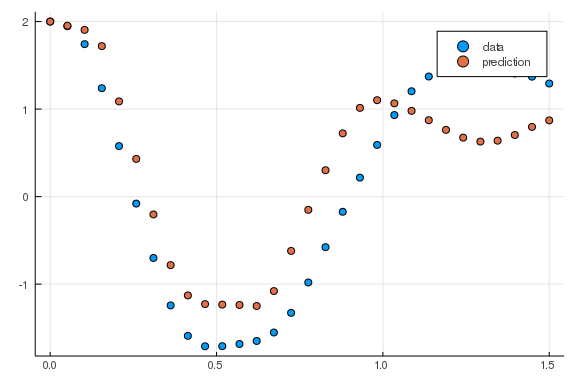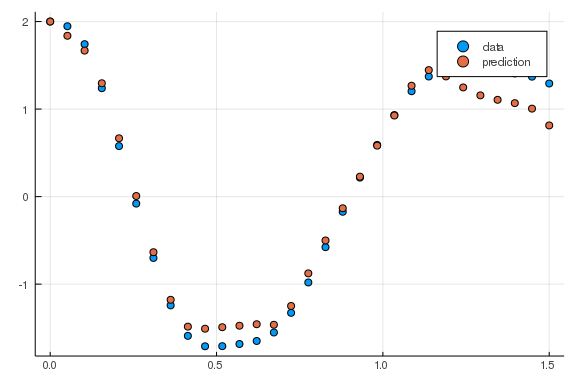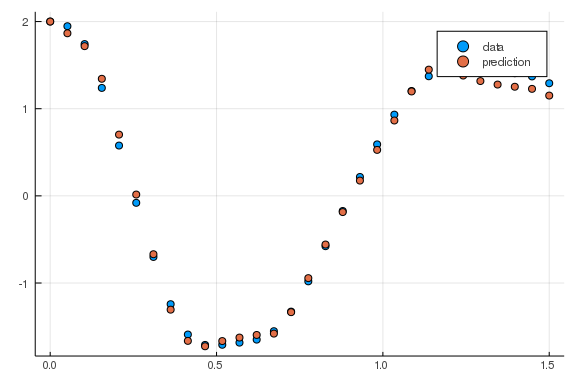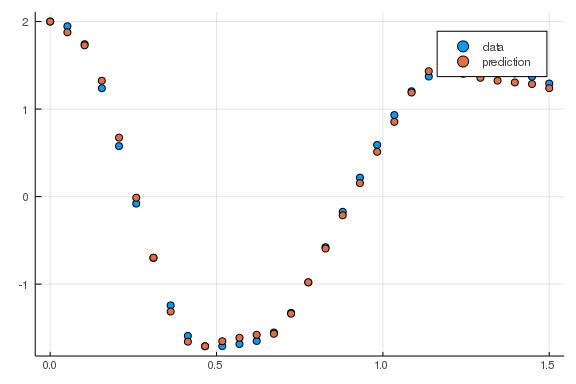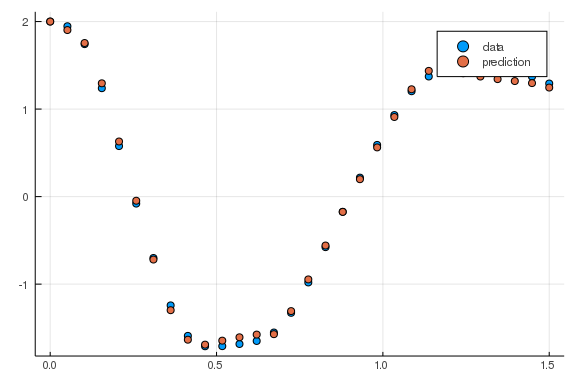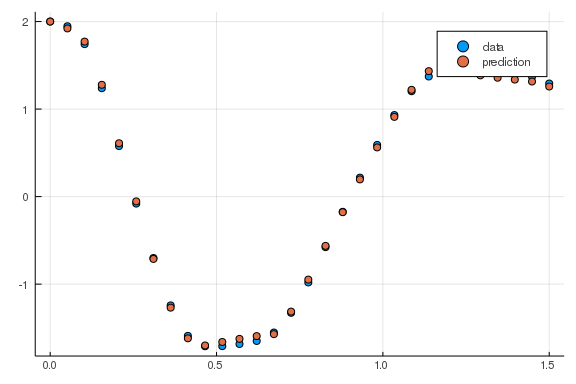### The Augmented Neural Ordinary Differential Equation

Note that not every function can be represented by an ordinary differential equation. Specifically, $u(t)$ is an $\mathbb{R} \rightarrow \mathbb{R}^n$ function which cannot loop over itself except when the solution is cyclic. The reason is because the flow of the ODE's solution is unique from every time point, and for it to have "two directions" at a point $u_i$ in phase space would have two solutions to the problem

$u' = f(u,p,t)$

where $u(0)=u_i$, and thus this cannot happen (with $f$ sufficiently nice). However, if we have another degree of freedom we can ensure that the ODE does not overlap with itself. This is the augmented neural ordinary differential equation.

We only need one degree of freedom in order to not collide, so we can do the following. We can add a fake state to the ODE which is zero at every single data point. This then allows this extra dimension to "bump around" as neccessary to let the function be a universal approximator. In code this looks like:

dudt = Chain(...)
p,re = Flux.destructure(dudt)
dudt_(u,p,t) = re(p)(u)
prob = ODEProblem(dudt_,[u0,0f0],tspan,p)
augmented_data = vcat(ode_data,zeros(1,size(ode_data,2)))


### The Universal Ordinary Differential Equation

This formulation of the nueral differential equation in terms of a "knowledge-embedded" structure is leading. If we already knew something about the differential equation, could we use that information in the differential equation definition itself? This leads us to the idea of the universal differential equation, which is a differential equation that embeds universal approximators in its definition to allow for learning arbitrary functions as pieces of the differential equation.

The best way to describe this object is to code up an example. As our example, let's say that we have a two-state system and know that the second state is defined by a linear ODE. This mean we want to write:

$x' = NN(x,y)$

$y' = p_1 x + p_2 y$

We can code this up as follows:

u0 = Float32[0.8; 0.8]
tspan = (0.0f0,25.0f0)

ann = Chain(Dense(2,10,tanh), Dense(10,1))

p1,re = Flux.destructure(ann)
p2 = Float32[-2.0,1.1]
p3 = [p1;p2]
ps = Flux.params(p3)

function dudt_(du,u,p,t)
x, y = u
du = re(p[1:41])(u)
du = p[end-1]*y + p[end]*x
end
prob = ODEProblem(dudt_,u0,tspan,p3)
concrete_solve(prob,Tsit5(),u0,p3,abstol=1e-8,reltol=1e-6)

t: 62-element Array{Float32,1}:
0.0
0.025993822
0.052559864
0.093415394
0.14211097
0.20731054
0.28383148
0.3714279
0.4711299
0.57710654
⋮
15.756952
16.553768
17.419676
18.367874
19.413296
20.576166
21.882597
23.367315
25.0
u: 62-element Array{Array{Float32,1},1}:
[0.8, 0.8]
[0.7885468, 0.7816014]
[0.77694905, 0.76343244]
[0.75932986, 0.7366743]
[0.7386807, 0.70653033]
[0.7116459, 0.6688899]
[0.6808314, 0.6282734]
[0.64679, 0.5859259]
[0.609661, 0.5423902]
[0.572082, 0.5007786]
⋮
[2.3593026e-5, 1.950222e-5]
[1.3841586e-5, 1.14415625e-5]
[7.7536815e-6, 6.4092533e-6]
[4.110698e-6, 3.397945e-6]
[2.0420862e-6, 1.688005e-6]
[9.378122e-7, 7.7520156e-7]
[3.9127758e-7, 3.2343414e-7]
[1.4493945e-7, 1.1980688e-7]
[4.8657576e-8, 4.0219856e-8]


and we can train the system to be stable at 1 as follows:

function predict_adjoint()
Array(concrete_solve(prob,Tsit5(),u0,p3,saveat=0.0:0.1:25.0))
end

data = Iterators.repeated((), 300)
iter = 0
cb = function ()
global iter += 1
if iter % 50 == 0
display(plot(solve(remake(prob,p=p3,u0=u0),Tsit5(),saveat=0.1),ylim=(0,6)))
end
end

# Display the ODE with the current parameter values.
cb()

Flux.train!(loss_adjoint, ps, data, opt, cb = cb)

235.02144f0
38.82619f0
25.955505f0
16.919544f0
10.137426f0
5.733651f0